### Introduction to Queues

A queue is an ordered collection of data items such that:

• the queue has two ends the front and the back

• the data items can be removed from only one end (i.e. the front of the queue)

• the data items can be added to only one end (i.e. the back of the queue)

A queue's basic operations are:

• construct: Creates an empty queue.

• isEmpty(): Checks to see if the queue is empty.

• isFull(): Checks to see if the queue is full.

• addQ(): Adds a data item to the back of the queue.

• front(): Retrieves the value of the data item from the front of the queue.

• removeQ(): Removes the data item at the front of the queue.

Because of its general construct, a queue is said to be a First-In-First-Out (FIFO) structure. In addition to being called FIFO, a queue is also called First-Come-First-Served (FCFS).

Note:  In some implementations the method: addQ() is called: enqueue(). Further in these implementations front() and removeQ() are combined into a method: dequeue() which returns the value at the front of the queue and then removes that value. However in these notes these methods will remain separate because some implementations of queues require the program to call front() to get its value, send this value to another site and then if the other site receives the value correctly, the queue is notified to remove the front element using the removeQ() method.

### Queues as Compared to Stacks

When compared as containers:

• Stacks are a LIFO container => they serve data in the reverse of order received

• Queues are a FIFO container => they serve data in the order received

When compared as to how they handle applications:

• Stacks are for applications where some sort of reversal or unwinding is desired.

• Queues are for applications where service is to be rendered relative to order received.

When these containers are used:

• Stacks and Queues can be used in conjunction to compare different orderings of the same data set.

From an ordering perspective, then, queues are the "opposite" of stacks.

### The Queue ADT Implemented Using an Array

Any implementation of a queue requires:

• storage for the data in a block of memory

• markers to the front and to the back of the queue.

Therefore an array-based implementation would need data constructs like:

• myArray, an array to store the elements of the queue

• myFront, an index to track the front queue element

• myBack, an index to track the position following last queue element

Problem:

Consider the following graphic as an example of an array implemented queue (notice that the capacity of the myArray is 11).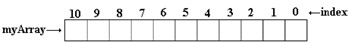To begin with, both myFront = 0 and myBack = 0. Any additions to the queue would be at the back and therefore the additions would result in incrementing myBack.

Note:  In the following discussion, each ai is a data item in myArray.

Add an element a1 to the queue so myBack = myBack + 1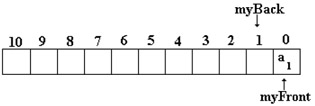Add another element a2 so myBack = myBack + 1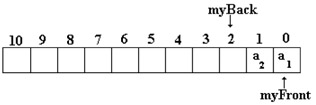Add another element a3 so myBack = myBack + 1 and then add another element a4 which yields myBack = myBack + 1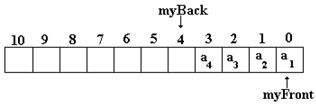Deletions from the front of the queue would result in incrementing myFront, so we have: myFront = myFront + 1 and the first element appears to be a2. However, if you looked in the memory, you would find a1 still in position 0. It is that the queue does not know that a1 is there. As far as the queue is concerned, the data in this position is garbage.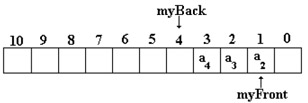If the additional elements: a5, a6, a7, a8, a9 and a10 are added to the queue then the code would need to increment myBack, once for each of these elements. In addition, if another element is removed from the queue myFront would be incremented as well and what would appear in the queue would be the following: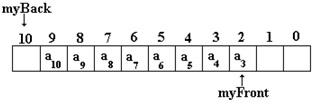Using a traditional approach to an array, as more data is added to the array, the array could run out of space soon! That is, if the program added a11, the counter: myBack would be incremented to exceed the size of the array. However this would not need to be the case in a queue if the following example was used: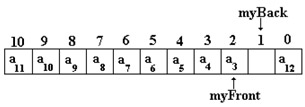In this example, you will notice that when a11 was added to the queue, the counter myBack took on the value 0 again and when a12 was added to the queue, myBack was incremented to 1. In a similar fashion when myFront reaches the end of the array, it too is set to 0 again.

Solution:

• Viewing this type of construction, the array is acting as a circular buffer, i.e. wrapping the end to the front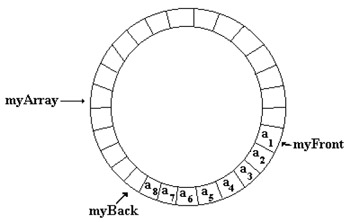Initially, a queue object is empty and the queue counters will have the following values:

• myFront = = 0

• myBack = = 0

After many insertions and deletions, the queue is full:

• First element, say, at myArray[i] and myFront has a value of i.

• Last element then at myArray[i-1] (i > 0) and myBack has a value of i.

PROBLEM: How to distinguish between empty & full?

Possible Solutions:

• Keep an empty slot between myFront and myBack, (i.e. myArray is allocated to capacity + 1 elements)

• Keep an auxiliary counter to track the actual number of elements in the queue

Wrap around keeps the addition/deletion cycle from walking off the edge of the storage array.

Say, myArray has capacity elements. When myBack hits the end of myArray, an addition should wrap myBack around to the first element of myArray, e.g.:` myBack++; if (myBack = = capacity)    myBack = 0; myBack = (myBack + 1) % capacity; `An analogous handling of myFront is needed when there is a deletion: e.g.` myFront = (myFront + 1) % capacity; `### An Example: A Queue as a Long Array

Create a C++ program that simulates the circle queue example above into a program. Set the array elements to be long integers. In doing this, the top and the bottom of the queue begin at position 0 because an array in C++ starts with index 0. Notice the following graphic which shows a circular queue with eight elements: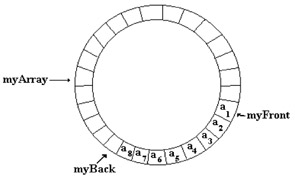Note:  An array is not the only way to solve this specification as will be discussed later in the lectures when Lists are discussed and when the STL (Standard Temple Library) is discussed.

#### The 5 Phases of Software Development

1. The Specification Phase

2. The Design Phase

3. The Coding Phase

• long_queue.h

• long_queue.cpp

• useQueue.cpp

(Are there cases where the program could fail? Has the program adequately provided for error handling? What should be added to ensure NO failure?)

4. The Testing Phase (What should be done here?)

5. The Maintenance Phase (What should be done here?)

Warning:  The Maintenance Phase should consider what the potential future changes can be. What the programmer needs to be very careful not to implement into the program what he/she believes will solve maintenance work of the future because it may never happen and these additions may make a program that the user does not want.

#### The Specifications Phase

Create a long queue. The queue should operate like the following example: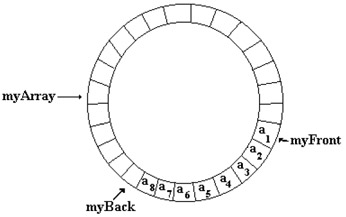The queue class needs to have methods that provide for each of the following:

• Construct a queue

• Check to determine whether the queue is empty

• Check to determine whether the queue is full

• Add an element at the back of the queue

• Retrieve the front element from the top of the queue

• Remove the front element of the queue

• Displays all of the elements of the queue (Normally this is not a method of a queue but it can be used here for testing.)

First consider some actions with the circle queue. For example:

• Create the queue

• View Top Element

• Remove

In the example above as elements are added: myFront is the index of the first element in the queue array while myBack is one position beyond the last element of the queue (i.e. where the next element can be added). However both myFront and myBack must rap around the array when they have reached the end of the array.

#### The Design Phase

To implement the specification, the program will create a C++ class: Queue. To do this we must determine the data members, the operations (member functions) and any other conditions that will be required to achieve the specifications. In addition we must always consider those cases that will make the operations fail. We should try to prevent any errors within the class if possible. If this is not possible, we need to consider what needs to be done in the implementation program to prevent ALL possible errors.

Independent of the class there will need to be a long:

• capacity: The size of the queue.

The class will have three data members:

 myFront: A long that is the index of location of the first element of the queue. myBack A long that is the location of one position beyond the last element added to the queue. theArray[ ] A long array that holds the elements of the queue.

The class methods that are required and the error considerations are the following:

• Construction: Creates the empty queue and initializes it by assigning values to myFront and myBack.

• isEmptyQ(): A method that determines if the queue contains any values by comparing whether the value of myFront and myBack are the same.

• isFullQ(): A method that determines if the queue has any more room for additional values by comparing the location of myFront in relationship to myBack so that there is always 1 space between them.

• addQ(): A method that modifies the queue by adding a value to the back of the queue and increments myBack so that it will rap around theArray[ ] if necessary thereby not to exceed the limit of theArray[ ]. Before any element is added it will be necessary to check to see if there is room in the queue for any more elements.

• topQ(): A method that retrieves the value at the front of the queue at location myFront provided that there are elements in the queue.

• removeQ(): A method that modifies a queue by removing the top value of the queue by incrementing myFront provided that there are elements to be removed from the queue.

To help with debugging, we will add the operation:

• displayQ(): A method that displays of all the elements stored in the queue. The display will have to begin where myFront is located and increment until myBack is reached. Should Display() reach the end of theArray[ ] before myBack is encountered, the operation will have to rap around to be at the beginning of the array.

The following would be an example of a UML diagram for the class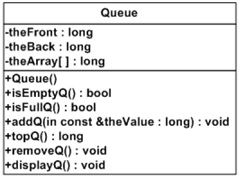As with the stack design phase above, the structure chart will not be discussed.

#### The Testing Phase

When designing the Testing Phase we need to keep in mind: The Program Must Never Fail No Matter What the User Does!!!! If this is our goal, then what should we do to test the program if we were a member of the Quality Assurance group? What types of errors could occur?

Some considerations to test:

• Test empty, full, display and remove when no elements have been added.

• Enter the following values: 80, 95, 77, 121 , 64, 234, 51, 29, 444

• Test empty, full and display if the elements have been added and if the operations are working correctly.

• Remove two elements and then test empty, full and display to see if the operations are working correctly.

• Enter the following values: 1428, 5432.

• Display to determine if everything is working correctly.

• The queue should now be full. Enter the 5643 to see if the program fails.

• Display to determine if it works correctly.

Can you think of any other possible tests? There is an error in the coding that is not well handled. Find it.

### Designing a General Queue Class

As with Stack, we will need to generalize the data type so that Queue can handle a long or a char or even a Date. To demonstrate the generality of this header we will use as the data type the class: Date which is in the header: date.h

What we will do now is to redesign the previous example so that the data type of the elements in Queue will not matter. This change will require that the data type of theArray[ ] in the previous example be specified outside of the definition of Queue in the implementation program. As with Stack, we again need to use a typedef in the implementation program as in the following statement:` typedef Date QueueType; `This will be done after the include of the Date header file. Of course we would change this if we wanted the data type of Queue to be something other than Date objects.

Next we need to change the definition of theArray[ ] as in the following statement:` QueueType theArray[capacity]; `In addition to this change of the array, it will be necessary to rewrite the prototypes and the definitions of two methods to reflect this change in data type of the queue. That is the following two functions must change:` void addQ(const QueueType& value); QueueType frontQ () const; `The redefinition of the class: Queue can be viewed in the header: new_queue.h.

Because of these changes, the implementation program must be changed in two ways:

• to reflection the changes in a general class Queue

• to reflect that the data elements in Queue are now objects of the class: Date found in the file: date.h

The implementation is now changed to the program: date_queue.cpp. This particular implementation is designed specifically for the class Date but with only minor changes it should work for any class.